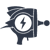Coming from the US, I have a pretty simplistic model of how postcodes work. They’re either 5 or 9 digits, depending on how precise you’re being. I can validate that with a simple regular expression. There’s a lot of detail encoded in that number, including the central sorting facility and city or town I’m in, but I don’t usually care about those details. In any country with some sort of postcode system, while the pattern of the postcode may change, the complexity of it generally caps out pretty quickly. That’s sort of the point.

Maybe Sam’s co-worker didn’t know what regexes were. Maybe they’d read an article talking about how regexes were expensive, and were worried about performance. Maybe they wrote this method as part of a college assignment and added it to their personal snippet library and never thought about it again. Maybe they just hated their co-workers and were planting landmines for them to stumble across. Regardless, this is how they verified a postcode within their locale.

``````public bool checkPostCode(string Postcode)
{
if (Postcode.Length < 9 && Postcode.Length > 5)
{
char[] array = Postcode.ToCharArray();
int l = array.Length;
bool end = false, start = false;

if ((int)Convert.ToChar(array[l - 1]) <= 90 && (int)Convert.ToChar(array[l - 1]) >= 65)
if ((int)Convert.ToChar(array[l - 2]) <= 90 && (int)Convert.ToChar(array[l - 2]) >= 65)
if ((int)Convert.ToChar(array[l - 3]) <= 57 && (int)Convert.ToChar(array[l - 3]) >= 48)
if ((int)Convert.ToChar(array[l - 4]) == 32)
end = true;//return true;

if (l == 6)
if ((int)Convert.ToChar(array) <= 90 && (int)Convert.ToChar(array) >= 65)
if (array <= 57 && array >= 48)
start = true;

if (l == 7)
{
if ((int)Convert.ToChar(array) <= 90 && (int)Convert.ToChar(array) >= 65)
{
if ((int)Convert.ToChar(array) <= 57 && (int)Convert.ToChar(array) >= 48)
if (((int)Convert.ToChar(array) <= 90 && (int)Convert.ToChar(array) >= 65) || ((int)Convert.ToChar(array) <= 57 && (int)Convert.ToChar(array) >= 48))
start = true;

if ((int)Convert.ToChar(array) <= 90 && (int)Convert.ToChar(array) >= 65)
if ((int)Convert.ToChar(array) <= 57 && (int)Convert.ToChar(array) >= 48)
start = true;
}
}

if (l == 8)
{
if ((int)Convert.ToChar(array) <= 90 && (int)Convert.ToChar(array) >= 65)
if ((int)Convert.ToChar(array) <= 90 && (int)Convert.ToChar(array) >= 65)
if ((int)Convert.ToChar(array) <= 57 && (int)Convert.ToChar(array) >= 48)
if (((int)Convert.ToChar(array) <= 90 && (int)Convert.ToChar(array) >= 65) || ((int)Convert.ToChar(array) <= 57 && (int)Convert.ToChar(array) >= 48))
start = true;
}

return start && end;
}
else
{
return false;
}
}``````

Well, that’s a thing. I really like how the first check cycles backwards through the string. I have no idea what locale is being targeted by this code, and I’m not about to trace through it to find out. Instead, I’ll just draw your attention to the `//return true` commented out, which gives us a sense of the mindset of the developer responsible for this. The whole thing says, “hope you have the ASCII table memorized, sucker”.[Advertisement] Forget logs. Next time you're struggling to replicate error, crash and performance issues in your apps - Think Raygun! Installs in minutes. Learn more.